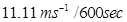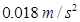### Motion - Solutions

CBSE class IX Science

NCERT Solutions
Chapter - 8
Motion

(Page No. 100)

1. An object has moved through a distance. Can it have zero displacement? If yes, support your answer with an example.

Ans. Yes, if an object has moved through a distance it can have zero displacement because displacement of an object is the actual change in its position when it moves from one position to the other. So if an object travels from point A to B and then returns back to point A again, the total displacement is zero.

2. A farmer moves along the boundary of a square field of side 10 m in 40 s. What will be the magnitude of displacement of the farmer at the end of 2 minutes 20 seconds?

Ans. Distance covered by farmer in 40 seconds = (40 x 1)m = 40 m

Speed of the farmer = distance/time = 40m/40s = 1 m/s.

Total time given in the question = 2min 20seconds = 60+60+20 =140 seconds

Since he completes 1round of the field in 40seconds so in he will complete 3 rounds in 120seconds (2mins) or 120m distance is covered in 2minutes. In another 20 seconds will cover another 20m so total distance covered in 2min20sec = 120 +20 =140m.

Displacement = $=\sqrt{{10}^{2}+{10}^{2}}$ = $\sqrt{200}$(as per diagram) = 10$\sqrt{2}$= 10 x 1.414 = 14.14 m.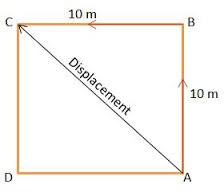3. Which of the following is true for displacement?

(a) It cannot be zero.
(b) Its magnitude is greater than the distance travelled by the object.

Ans. Both (a) as well as (b) are false with respect to concept of displacement.

(Page No. 102)

1. Distinguish between speed and velocity.

Ans. Speed of a body is the distance travelled by it per unit time while velocity is displacement per unit time of the body during movement.

2. Under what condition(s) is the magnitude of average velocity of an object equal to its average speed?

Ans. If distance travelled by an object is equal to its displacement then the magnitude of average velocity of an object will be equal to its average speed.

3. What does the odometer of an automobile measure?

Ans. The odometer of an automobile measures the distance covered by that automobile.

4. What does the path of an object look like when it is in uniform motion?

Ans. Graphically the path of an object will be linear i.e. looks like a straight line when it is in uniform motion.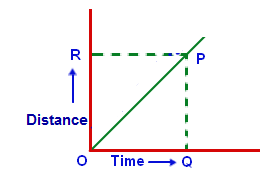5. During an experiment, a signal from a spaceship reached the ground station in five minutes. What was the distance of the spaceship from the ground station? The signal travels at the speed of light, that is,3 x 108 ms-1.

Ans. Distance = speed x time = 5min x (3 x 108ms-1) = (5 x 60)second x (3 x 108ms-1)
= 300sec x (3 x 108ms-1)
= 900 x 108ms-1 = 9 x 1010m
= 9 x 107km

(Page No. 103)

1. When will you say a body is in

(i) uniform acceleration?
(ii) non-uniform acceleration?

Ans. (i) uniform acceleration: When an object travels in a straight line and its velocity changes by equal amount in equal intervals of time, it is said to have uniform acceleration.

(ii) non uniform acceleration: It is also called variable acceleration. When the velocity of an object changes by unequal amounts in equal intervals of time, it is said to have non uniform acceleration.

2. A bus decreases its speed from 80 km h-1 to 60 km h-1 in 5 s. Find the acceleration of the bus.

Ans. Initial speed of bus (u) = 80kmh-1 = 80 x 1000/60x60 seconds
= 200/9ms-1 = 22.22ms-1
final speed of bus (v)= 60km h-1= 60 x 1000/60 x 60 seconds
= 50/3ms-1= 16.67ms-1 time (t) = 5 s
acceleration (a) = ( v – u) /t = (16.67 – 22.22)/5 = -5.55/5 = -1.11 m/s2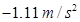3. A train starting from a railway station and moving with uniform acceleration attains a speed 40km h-1 in 10 minutes. Find its acceleration.

Ans. Since the train starts from rest(railway station) = u = zero
Final velocity of train =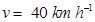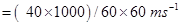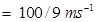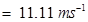time (t) = 10 min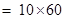= 600 seconds

Since a = (v – u )/t =